Anúncio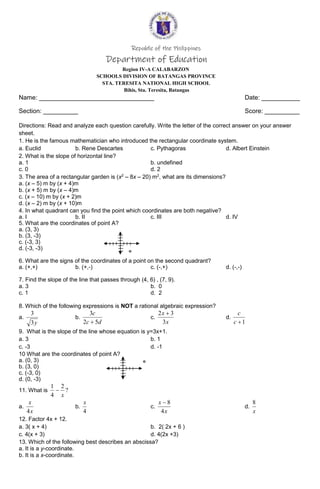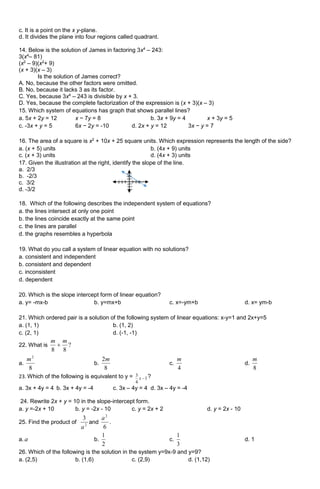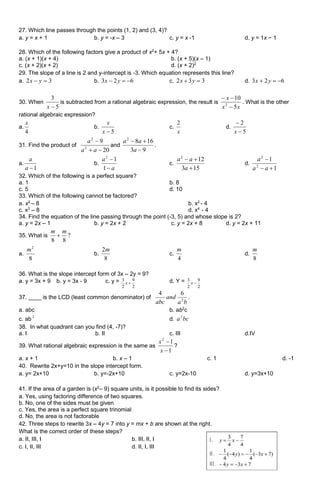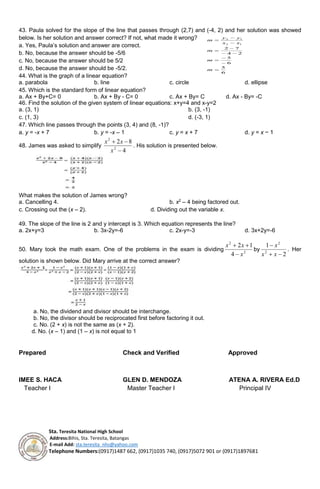Anúncio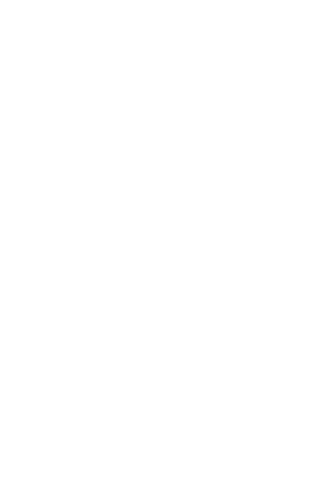Próximos SlideShares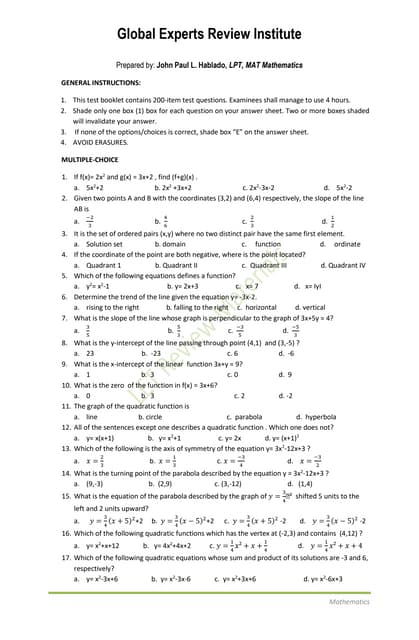Math exercises
Carregando em ... 3
1 de 5
Anúncio

### 1st-PT-8 (2).doc

1. Republic of the Philippines Department of Education Region IV-A CALABARZON SCHOOLS DIVISION OF BATANGAS PROVINCE STA. TERESITA NATIONAL HIGH SCHOOL Bihis, Sta. Teresita, Batangas Name: _________________________________ Date: ___________ Section: __________ Score: __________ Directions: Read and analyze each question carefully. Write the letter of the correct answer on your answer sheet. 1. He is the famous mathematician who introduced the rectangular coordinate system. a. Euclid b. Rene Descartes c. Pythagoras d. Albert Einstein 2. What is the slope of horizontal line? a. 1 b. undefined c. 0 d. 2 3. The area of a rectangular garden is (x2 – 8x – 20) m2 , what are its dimensions? a. (x – 5) m by (x + 4)m b. (x + 5) m by (x – 4)m c. (x – 10) m by (x + 2)m d. (x – 2) m by (x + 10)m 4. In what quadrant can you find the point which coordinates are both negative? a. I b. II c. III d. IV 5. What are the coordinates of point A? a. (3, 3) b. (3, -3) c. (-3, 3) d. (-3, -3) 6. What are the signs of the coordinates of a point on the second quadrant? a. (+,+) b. (+,-) c. (-,+) d. (-,-) 7. Find the slope of the line that passes through (4, 6) , (7, 9). a. 3 b. 0 c. 1 d. 2 8. Which of the following expressions is NOT a rational algebraic expression? a. y 3 3 b. d c c 5 2 3  c. x x 3 3 2  d. 1  c c 9. What is the slope of the line whose equation is y=3x+1. a. 3 b. 1 c. -3 d. -1 10 What are the coordinates of point A? a. (0, 3) b. (3, 0) c. (-3, 0) d. (0, -3) 11. What is ? 2 4 1 x  a. x x 4 b. 4 x c. x x 4 8  d. x 8 12. Factor 4x + 12. a. 3( x + 4) b. 2( 2x + 6 ) c. 4(x + 3) d. 4(2x +3) 13. Which of the following best describes an abscissa? a. It is a y-coordinate. b. It is a x-coordinate.
2. c. It is a point on the x y-plane. d. It divides the plane into four regions called quadrant. 14. Below is the solution of James in factoring 3x4 – 243: 3(x4 – 81) (x2 – 9)(x2 + 9) (x + 3)(x – 3) Is the solution of James correct? A. No, because the other factors were omitted. B. No, because it lacks 3 as its factor. C. Yes, because 3x4 – 243 is divisible by x + 3. D. Yes, because the complete factorization of the expression is (x + 3)(x – 3) 15. Which system of equations has graph that shows parallel lines? a. 5x + 2y = 12 x − 7y = 8 b. 3x + 9y = 4 x + 3y = 5 c. -3x + y = 5 6x − 2y = -10 d. 2x + y = 12 3x − y = 7 16. The area of a square is x2 + 10x + 25 square units. Which expression represents the length of the side? a. (x + 5) units b. (4x + 9) units c. (x + 3) units d. (4x + 3) units 17. Given the illustration at the right, identify the slope of the line. a. 2/3 b. -2/3 c. 3/2 d. -3/2 18. Which of the following describes the independent system of equations? a. the lines intersect at only one point b. the lines coincide exactly at the same point c. the lines are parallel d. the graphs resembles a hyperbola 19. What do you call a system of linear equation with no solutions? a. consistent and independent b. consistent and dependent c. inconsistent d. dependent 20. Which is the slope intercept form of linear equation? a. y= -mx-b b. y=mx+b c. x=-ym+b d. x= ym-b 21. Which ordered pair is a solution of the following system of linear equations: x-y=1 and 2x+y=5 a. (1, 1) b. (1, 2) c. (2, 1) d. (-1, -1) 22. What is ? 8 8 m m  a. 8 2 m b. 8 2m c. 4 m d. 8 m 23. Which of the following is equivalent to y = 1 4 3  x ? a. 3x + 4y = 4 b. 3x + 4y = -4 c. 3x – 4y = 4 d. 3x – 4y = -4 24. Rewrite 2x + y = 10 in the slope-intercept form. a. y =-2x + 10 b. y = -2x - 10 c. y = 2x + 2 d. y = 2x - 10 25. Find the product of 2 3 a and 6 2 a . a. a b. 2 1 c. 3 1 d. 1 26. Which of the following is the solution in the system y=9x-9 and y=9? a. (2,5) b. (1,6) c. (2,9) d. (1,12)
3. 27. Which line passes through the points (1, 2) and (3, 4)? a. y = x + 1 b. y = -x – 3 c. y = x -1 d. y = 1x − 1 28. Which of the following factors give a product of x2 + 5x + 4? a. (x + 1)(x + 4) b. (x + 5)(x – 1) c. (x + 2)(x + 2) d. (x + 2)2 29. The slope of a line is 2 and y-intercept is -3. Which equation represents this line? a. 3 2   y x b. 6 2 3    y x c. 3 3 2   y x d. 6 2 3    y x 30. When 5 3  x is subtracted from a rational algebraic expression, the result is x x x 5 10 2    . What is the other rational algebraic expression? a. 4 x b. 5  x x c. x 2 d. 5 2   x 31. Find the product of 20 9 2 2    a a a and 9 3 16 8 2    a a a . a. 1  a a b. a a   1 1 2 c. 15 3 12 2    a a a d. 1 1 2 2    a a a 32. Which of the following is a perfect square? a. 1 b. 8 c. 5 d. 10 33. Which of the following cannot be factored? a. x2 – 8 b. x2 - 4 c. x3 – 8 d. x4 - 4 34. Find the equation of the line passing through the point (-3, 5) and whose slope is 2? a. y = 2x – 1 b. y = 2x + 2 c. y = 2x + 8 d. y = 2x + 11 35. What is ? 8 8 m m  a. 8 2 m b. 8 2m c. 4 m d. 8 m 36. What is the slope intercept form of 3x – 2y = 9? a. y = 3x + 9 b. y = 3x - 9 c. y = 2 9 2 3  x d. Y = 2 9 2 3  x 37. ____ is the LCD (least common denominator) of b a and abc 2 6 4 . a. abc b. ab2 c c. ab 2 d. bc a2 38. In what quadrant can you find (4, -7)? a. I b. II c. III d.IV 39. What rational algebraic expression is the same as 1 1 2   x x ? a. x + 1 b. x – 1 c. 1 d. -1 40. Rewrite 2x+y=10 in the slope intercept form. a. y= 2x+10 b. y=-2x+10 c. y=2x-10 d. y=3x+10 41. If the area of a garden is (x2 – 9) square units, is it possible to find its sides? a. Yes, using factoring difference of two squares. b. No, one of the sides must be given c. Yes, the area is a perfect square trinomial d. No, the area is not factorable 42. Three steps to rewrite 3x – 4y = 7 into y = mx + b are shown at the right. What is the correct order of these steps? a. II, III, I b. III, II, I c. I, II, III d. II, I, III
4. 6 5 6 5 2 4 7 2 1 2 1 2            m m m x x y y m 43. Paula solved for the slope of the line that passes through (2,7) and (-4, 2) and her solution was showed below. Is her solution and answer correct? If not, what made it wrong? a. Yes, Paula’s solution and answer are correct. b. No, because the answer should be -5/6 c. No, because the answer should be 5/2 d. No, because the answer should be -5/2. 44. What is the graph of a linear equation? a. parabola b. line c. circle d. ellipse 45. Which is the standard form of linear equation? a. Ax + By+C= 0 b. Ax + By - C= 0 c. Ax + By= C d. Ax - By= -C 46. Find the solution of the given system of linear equations: x+y=4 and x-y=2 a. (3, 1) b. (3, -1) c. (1, 3) d. (-3, 1) 47. Which line passes through the points (3, 4) and (8, -1)? a. y = -x + 7 b. y = -x – 1 c. y = x + 7 d. y = x − 1 48. James was asked to simplify 4 8 2 2 2    x x x . His solution is presented below. What makes the solution of James wrong? a. Cancelling 4. b. x2 – 4 being factored out. c. Crossing out the (x – 2). d. Dividing out the variable x. 49. The slope of the line is 2 and y intercept is 3. Which equation represents the line? a. 2x+y=3 b. 3x-2y=-6 c. 2x-y=-3 d. 3x+2y=-6 50. Mary took the math exam. One of the problems in the exam is dividing 2 2 4 1 2 x x x    by 2 1 2 2    x x x . Her solution is shown below. Did Mary arrive at the correct answer? a. No, the dividend and divisor should be interchange. b. No, the divisor should be reciprocated first before factoring it out. c. No. (2 + x) is not the same as (x + 2). d. No. (x – 1) and (1 – x) is not equal to 1 Prepared Check and Verified Approved IMEE S. HACA GLEN D. MENDOZA ATENA A. RIVERA Ed.D Teacher I Master Teacher I Principal IV Sta. Teresita National High School Address:Bihis, Sta. Teresita, Batangas E-mail Add: sta.teresita_nhs@yahoo.com Telephone Numbers:(0917)1487 662, (0917)1035 740, (0917)5072 901 or (0917)1897681
Anúncio